# The Histogram Filter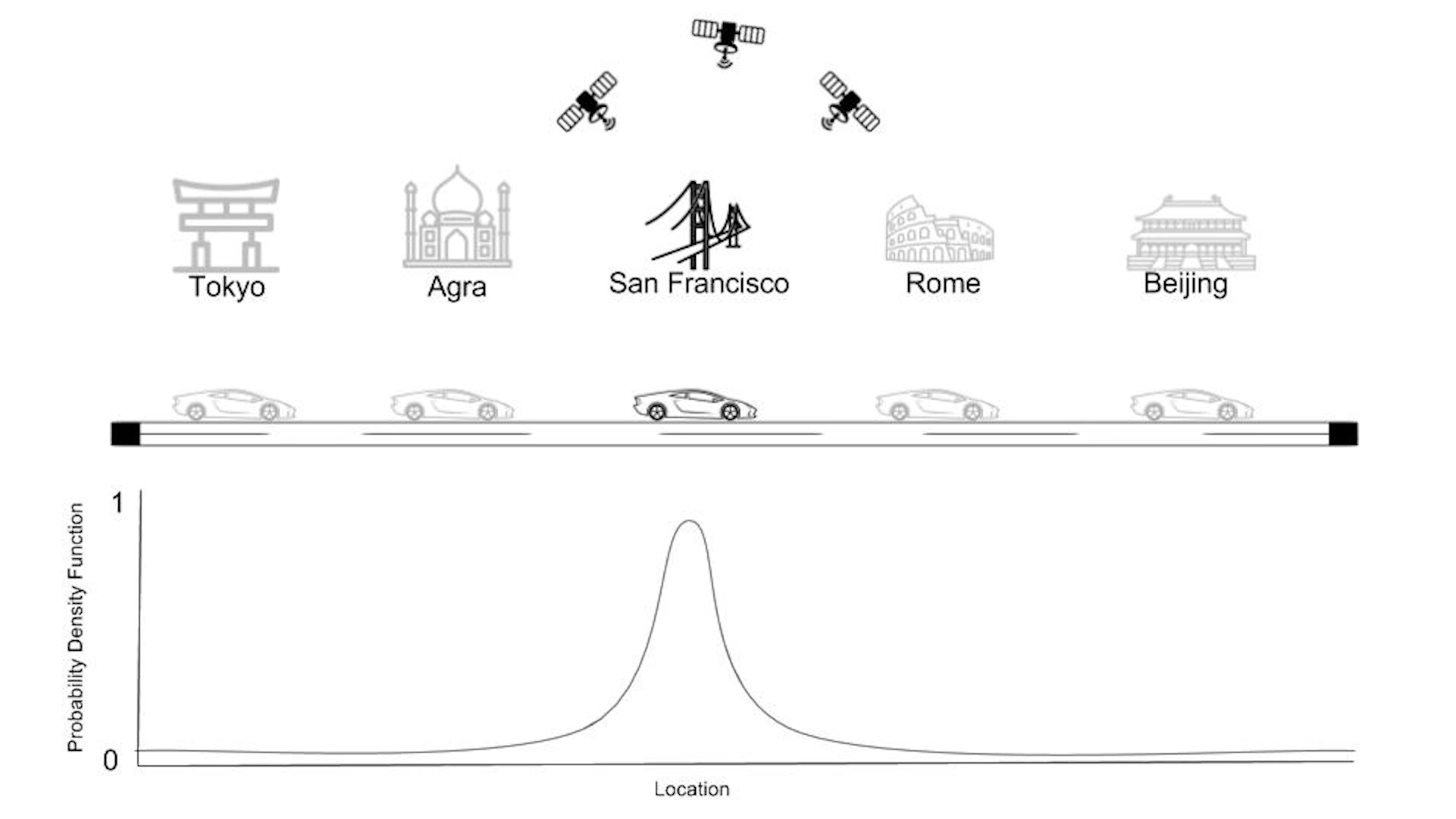Course Notes from Udacity Self-Driving Car Nanodegree Program

### 概率分布模型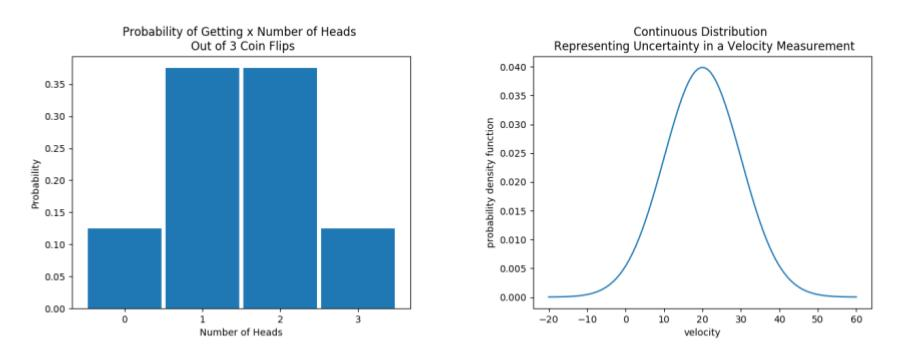• 对于离散概率分布函数g(x)，有如下性质
1. 对于所有的x值，对应的y值均大于等于0
2. 对于任意x值，其对应的y值表示该x事件发生的概率p(x)
3. 所有g(x)值的和为1
4. 对任意g(x)的值均小于等于1
• 对于连续概率分布函数f(x)，有如下性质
1. 对于所有的x值，对应的y值均大于等于0
2. 对于任意x值，它的概率为0
3. 对于某个区间内的event发生的概率为该区间的面积
4. f(x)的面积为1
5. 对任意f(x)的值可以大于1

## Localization的原理

### Sense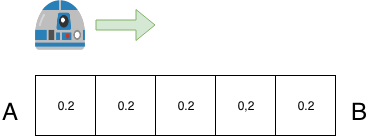p=[0.2,0.2,0.2,0.2,0.2]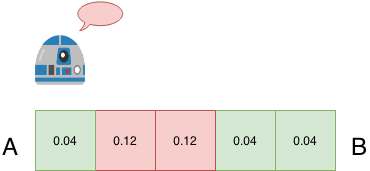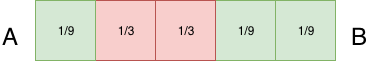p=[0.2, 0.2, 0.2, 0.2, 0.2] #先验概率
world=['green', 'red', 'red', 'green', 'green'] #格子颜色

#调整因子
pHit = 0.6
pMiss = 0.2

#Z为传感器残疾结果
def sense(p, Z):
q=[]
for i in range(len(p)):
hit = (Z == world[i])
## 贝叶斯公式，求乘积
q.append(p[i] * (hit * pHit + (1-hit) * pMiss))

#归一化
total = sum(q)
q = map(lambda x:x/total,q)

return q


sense函数非常重要，接下来会不断使用这个函数，因此一定要充分理解。回到上面的例子，当机器人sense到自己红色的时候，此时自己内部的概率分布发生了变化，从左图变成了右图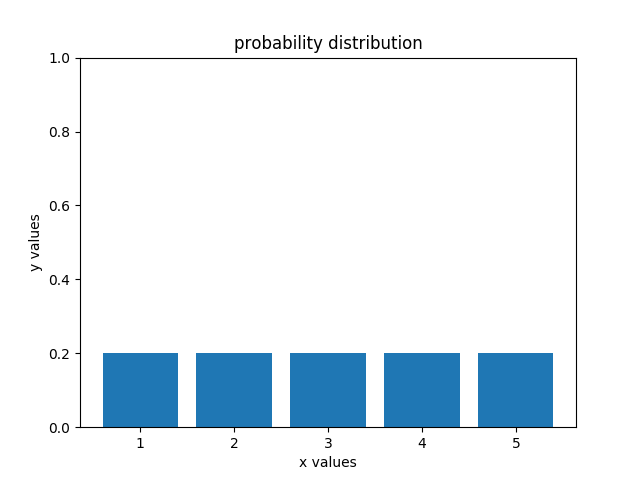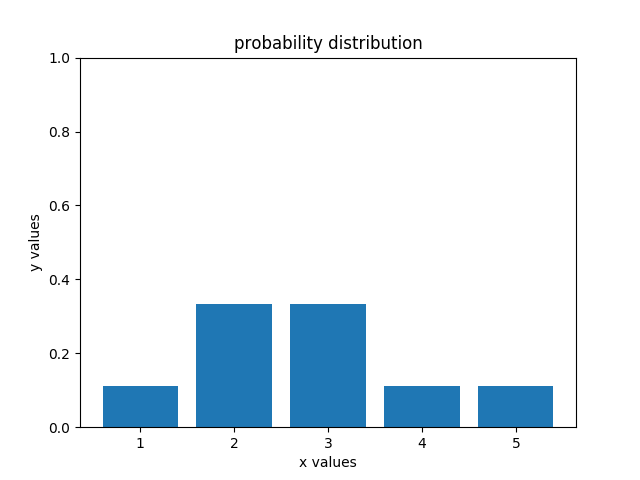p = move(p,1)
p = sense(p,'green')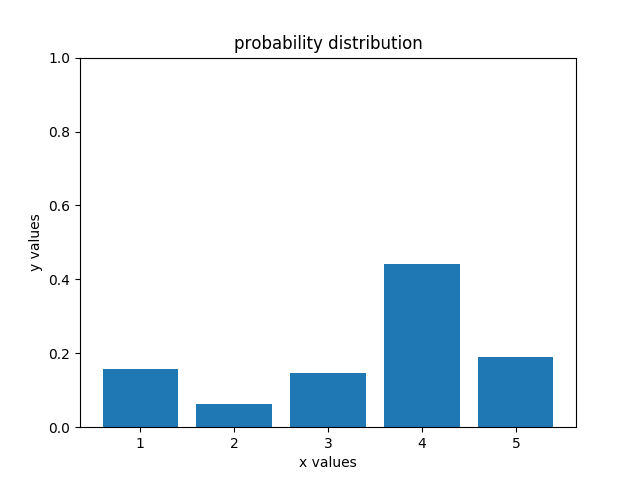p = sense(p,'red')
p = move(p,1)
p = sense(p,'green')
p = move(p,1)
...


### Move

p = [0,1,0,0,0]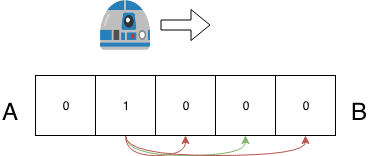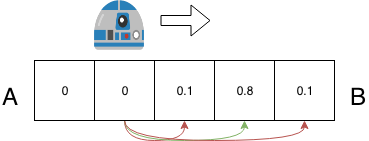p = [0,0,0.1,0.8,0.1]


def move(p, U):
q = []
for i in range(len(p)):
s = pExact * p[(i-U) % len(p)]
##计算全概率，求和
s = s + pOvershoot * p[(i-U-1) % len(p)]
s = s + pUndershoot * p[(i-U+1) % len(p)]
q.append(s)
return q

p=[1,0,0,0,0]

pExact = 0.8
pOvershoot = 0.1
pUndershoot = 0.1

for _ in range(1,10001):
p = move(p,1)

print(p) #[0.2,0.2,0.2,0.2,0.2]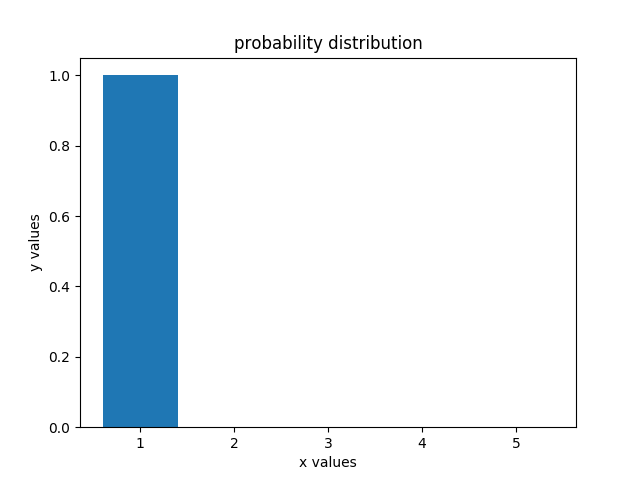### Sense and Move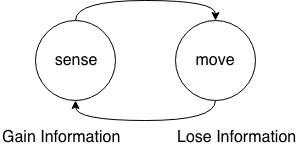p=[0.2, 0.2, 0.2, 0.2, 0.2]
world=['green', 'red', 'red', 'green', 'green']

measurements = ['red', 'green']
motions = [1,1]

for k in range(len(measurements)):
#sense
p = sense(p,measurements[k])
#move
p = move(p,motions[k])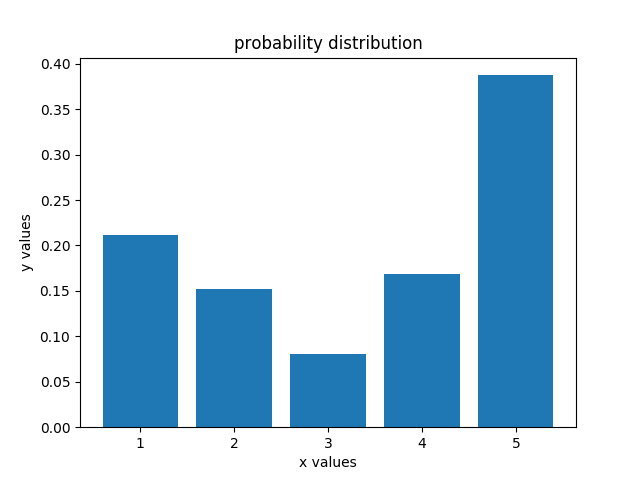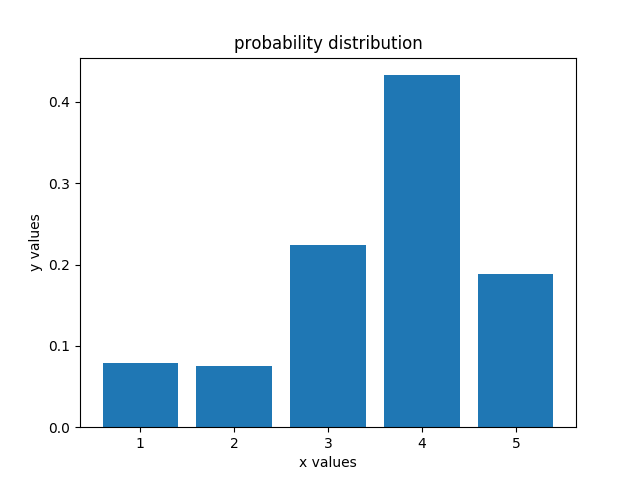### 小结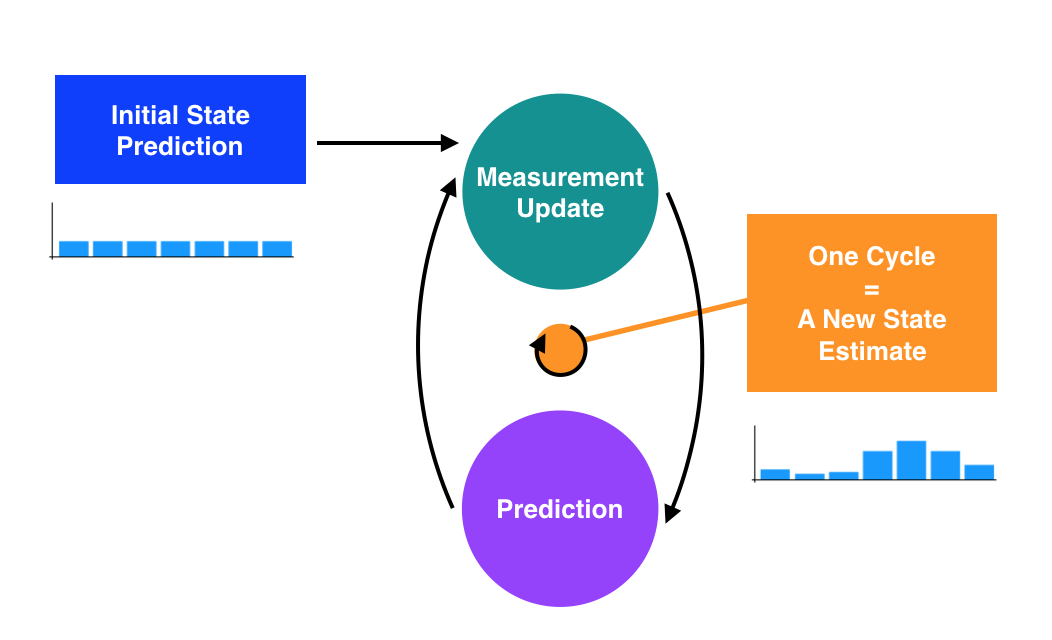## 一维卡尔曼滤波

### Measurement Update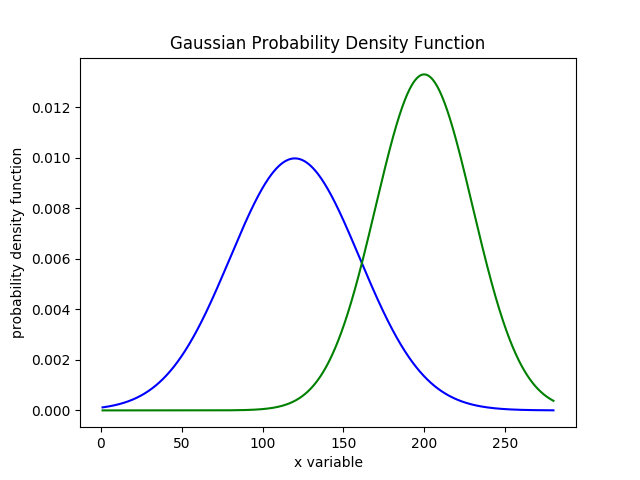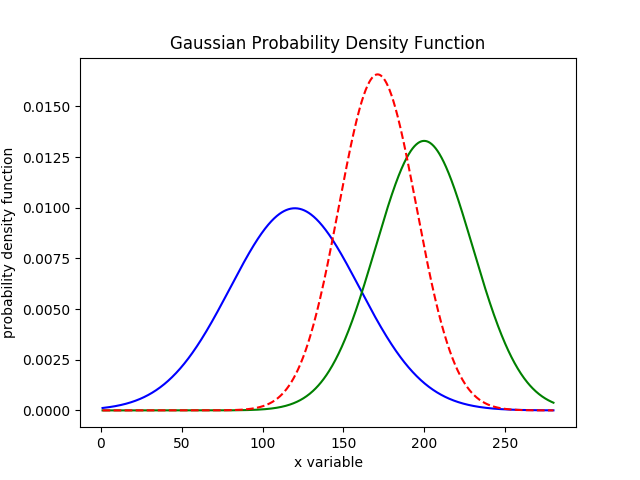1. 计算后的高斯分布的均值位于120200之间
2. 方差变的更小，峰值为三者最高，说明measure获取了新的信息，提高了位置预测准确率

measure过程的Python代码如下：

def measure(mean1, var1, mean2, var2):
new_mean = (var2 * mean1 + var1 * mean2) / (var1 + var2)
new_var = 1/(1/var1 + 1/var2)
return (new_mean, new_var)


### Motion Update

sense完之后就可以开始move了，move是一个损失信息的过程，为了量化损失的信息，我们引入一个损失概率密度函数，该函数也服从高斯分布，我们用(u,r)表示，其中u是均值，r是方差。为了计算损耗后的概率分布，我们只需要让当前的概率密度函数和损失概率密度函数“求和”即可：

def predict(mean1, var1, mean2, var2):
new_mean = mean1 + mean2
new_var = var1 + var2
return (new_mean, new_var)


### sense & move

import math
from Kalman.predict import predict
from Kalman.measure import measure

##先验概率
mu = 0
sig = 1000

##矫正因子
measurements = [5.0,6.0,7.0,9.0,10.0]
measurement_sig = 4.0

##损耗因子
motion = [1.0,1.0,2.0,1.0,1.0]
motion_sig = 2.0

## sense and move
for index in range(0,len(measurements)):
#sense
(mu,sig) = measure(mu,sig, measurements[index],measurement_sig)
#move
(mu,sig) = predict(mu,sig, motion[index],motion_sig)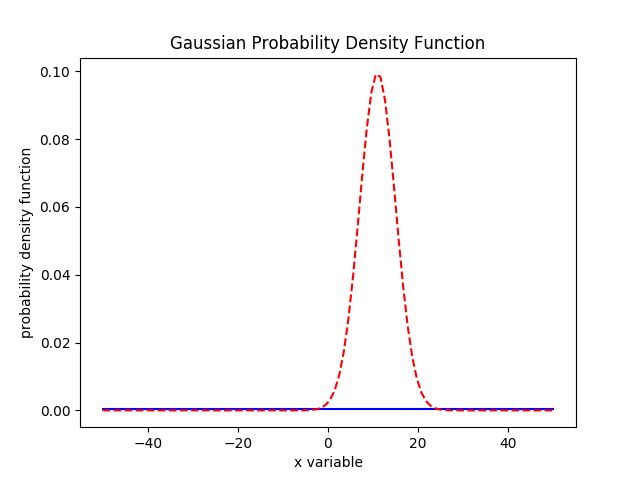（未完待续）